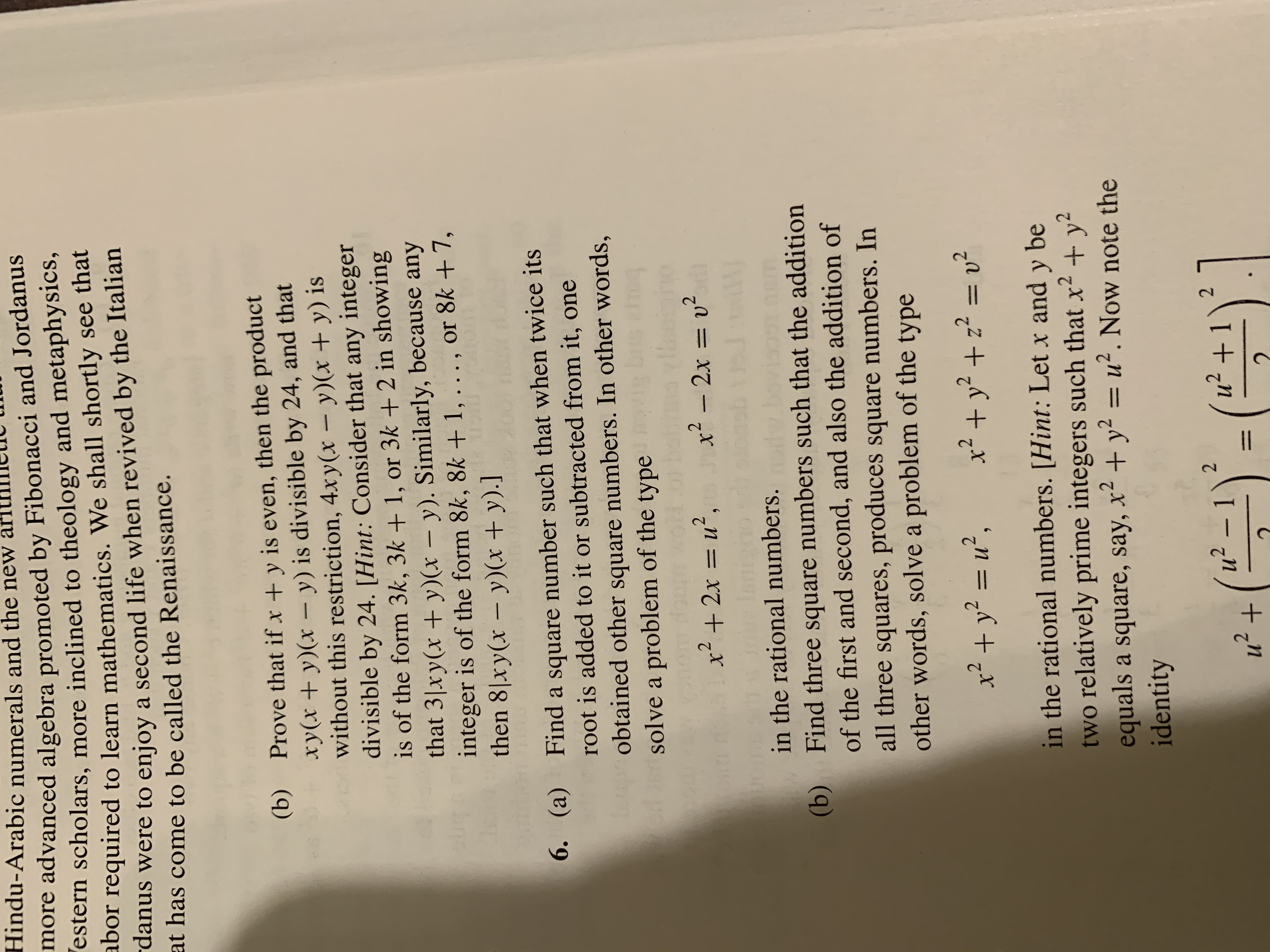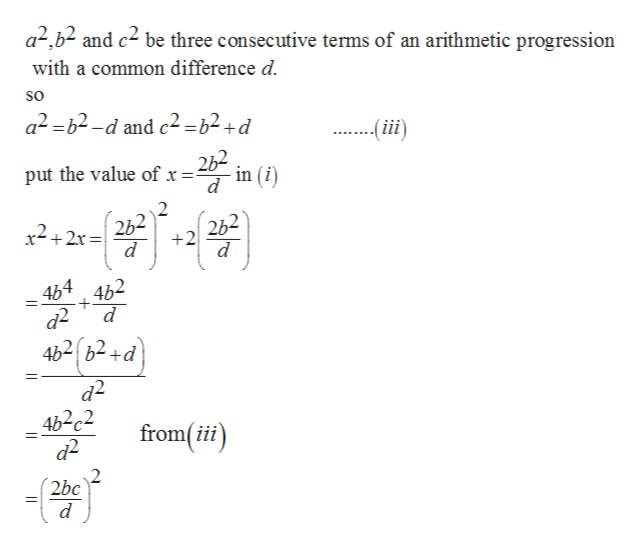# Hindu-Arabic numerals and the newmore advanced algebra promoted by Fibonacci and Jordanusestern scholars, more inclined to theology and metaphysics,abor required to learn mathematics. We shall shortly see thatdanus were to enjoy a second life when revived by the Italianat has come to be called the Renaissance.Prove that if x +y is even, then the productxy(x +y)(x - y) is divisible by 24, and thatwithout this restriction, 4xy(x - y)(x + y) isdivisible by 24. [Hint: Consider that any integeris of the form 3k, 3k + 1, or 3k + 2 in showingthat 3 xy(x +y)(x - y). Similarly, because anyinteger is of the form 8k, 8k + 1, . . . , or 8k + 7,then 8xy(x - y)(x + y).](b)bigFind a square number such that when twice itsroot is added to it or subtracted from it, one(a)6.obtained other square numbers. In other words,solve a problem of the typex2-2x = 2in the rational numbers.(b)Find three square numbers such that the additionof the first and second, and also the addition ofall three squares, produces square numbers. Inother words, solve a problem of the type2x +y22 v2in the rational numbers. [Hint: Let x and y betwo relatively prime integers such that x2 yequals a square, say, x2 +y2 u2. Now note theidentity22 -12u212

Question
11 viewshelp_outlineImage TranscriptioncloseHindu-Arabic numerals and the new more advanced algebra promoted by Fibonacci and Jordanus estern scholars, more inclined to theology and metaphysics, abor required to learn mathematics. We shall shortly see that danus were to enjoy a second life when revived by the Italian at has come to be called the Renaissance. Prove that if x +y is even, then the product xy(x +y)(x - y) is divisible by 24, and that without this restriction, 4xy(x - y)(x + y) is divisible by 24. [Hint: Consider that any integer is of the form 3k, 3k + 1, or 3k + 2 in showing that 3 xy(x +y)(x - y). Similarly, because any integer is of the form 8k, 8k + 1, . . . , or 8k + 7, then 8xy(x - y)(x + y).] (b) big Find a square number such that when twice its root is added to it or subtracted from it, one (a) 6. obtained other square numbers. In other words, solve a problem of the type x2-2x = 2 in the rational numbers. (b) Find three square numbers such that the addition of the first and second, and also the addition of all three squares, produces square numbers. In other words, solve a problem of the type 2 x +y22 v2 in the rational numbers. [Hint: Let x and y be two relatively prime integers such that x2 y equals a square, say, x2 +y2 u2. Now note the identity 2 2 -1 2 u21 2 fullscreen
check_circle

Step 1

To solve the given problem.

Step 2

Let us assume three sq...help_outlineImage Transcriptionclosea2b2 and c2 be three consecutive terms of an arithmetic progression with a common difference d so a2-b2-d and c2-b2+d -iii) put the value of x in (i d 2b2 262 +2 d d 464 462 d 4b2b2+d а2 4b2c2 2 from(ii) 2bc d fullscreen

### Want to see the full answer?

See Solution

#### Want to see this answer and more?

Solutions are written by subject experts who are available 24/7. Questions are typically answered within 1 hour.*

See Solution
*Response times may vary by subject and question.
Tagged in

### Math